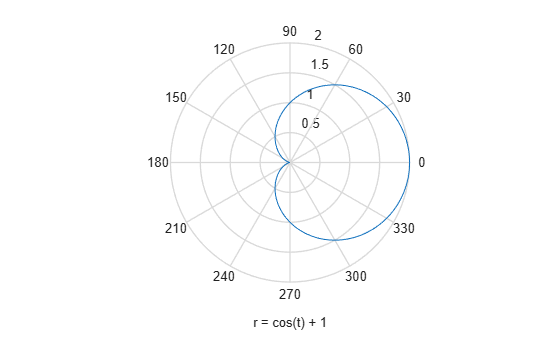# ezpolar

Polar coordinate plotter

## Syntax

``ezpolar(f)``
``ezpolar(f, [a b])``

## Description

example

````ezpolar(f)` plots the polar curve r = f(θ) over the default domain 0 < θ < 2π.```
````ezpolar(f, [a b])` plots f for `a` < θ < `b`.```

## Examples

collapse all

Create a polar plot of the function $r\left(t\right)=1+\mathrm{cos}\left(t\right)$ over the domain $0.

```syms t ezpolar(1 + cos(t))```## Input Arguments

collapse all

Input, specified as a symbolic function or expression.

Angle to plot over, specified as a vector, or a symbolic vector.

## Version History

Introduced before R2006a